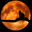Related Tags

pandas
python
data analysis
community creator

# How to delete a column in pandasNeko Yan

## Drop the column

DataFrame has a method called drop() that removes rows or columns according to specify column(label) names and corresponding axis.

import pandas as pd

# Create a dataframe from a dict
df = pd.DataFrame({"a": [1,2,3], "b":[2,4,6]})
print("The DataFrame object before deleting the column")
print(df)
df.drop('a', inplace=True, axis=1)
print("The DataFrame object after deleting the column a")
print(df)
Drop the column
• line 7 shows how to drop a column by calling drop. inplace=True means the operation would work on the original object. axis=1 means we are dropping the column, not the row.
• You can compare the output of line 6 and line 9.

## Delete the column

del is also an option, you can delete a column by del df['column name']. The Python would map this operation to df.__delitem__('column name'), which is an internal method of DataFrame.

import pandas as pd

# Create a dataframe from a dict
df = pd.DataFrame({"a": [1,2,3], "b":[2,4,6]})
print("The DataFrame object before deleting the column")
print(df)
del df["a"]
print("The DataFrame object after deleting the column a")
print(df)
Delete the column
• line 7 shows how to use del to delete a column from a DataFrame object.

## Pop the column

pop() function would also drop the column. Unlike the other two methods, this function would return the column.

import pandas as pd

# Create a dataframe from a dict
df = pd.DataFrame({"a": [1,2,3], "b":[2,4,6]})
print("The DataFrame object before deleting the column")
print(df)
df.pop("a")
print("The DataFrame object after deleting the column a")
print(df)
Pop the column
• line 7 shows how to use pop() to delete a column.

RELATED TAGS

pandas
python
data analysis
community creator

CONTRIBUTORNeko Yan
RELATED COURSES

View all Courses

Learn in-demand tech skills in half the time